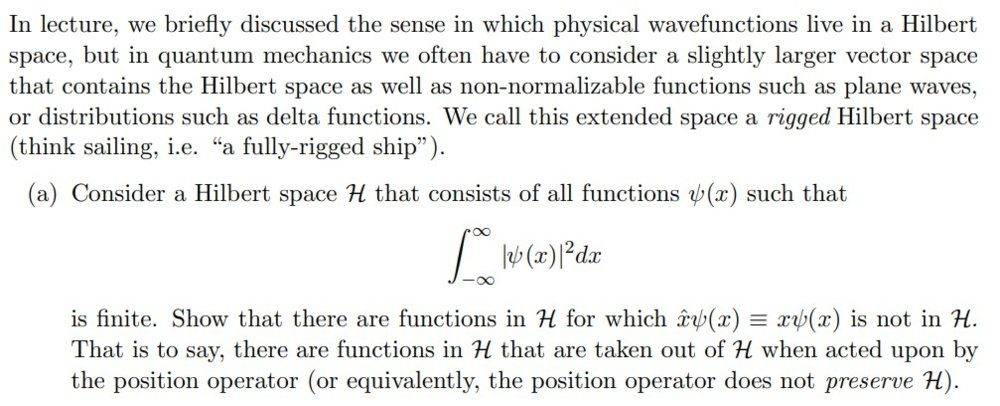# Show that the position operator does not preserve H

Moolisa
Homework Statement:
Consider a Hilbert space H that consists of all functions ψ(x) such that

##\int_{-\infty}^{\infty} |\psi(x)|^2, dx##

is finite. Show that there are functions in H for which ˆxψ(x) ≡ xψ(x) is not in H.
That is to say, there are functions in H that are taken out of H when acted upon by
the position operator (or equivalently, the position operator does not preserve H)
Relevant Equations:
##\int_-\infty^\infty |ψ(x)|^2\, dx##
ˆxψ(x) ≡ xψ(x)The attempt

##\int_{-\infty}^{\infty} |ψ^*(x)\, \hat x\,\psi(x)|\, dxˆ##

Using ˆxψ(x) ≡ xψ(x)

=##\int_{-\infty}^{\infty} |ψ^*(x)\,x\,\psi(x)|\, dxˆ##

=##\int_{-\infty}^{\infty} |ψ^*(x)\,\psi(x)\,x|\, dxˆ##

=##\int_{-\infty}^{\infty} |x\,ψ^2(x)|\, dxˆ##

I'm pretty sure this is not the correct approach. ψ(x) is a stationary right? Is it safe to attempt this using the definition of a stationary state? I apologize if I'm completely wrong, I've read the text and lecture notes but am still having a lot of trouble understanding this

Last edited:

Abhishek11235
If I parsed your question correctly,then think of this function:
##\psi=1/x##

Does this meets hypothesis?

Moolisa
If I parsed your question correctly,then think of this function:
##\psi=1/x##

Does this meets hypothesis?

I think so? If acted on by the position operator it would no longer be finite would it?

•Abhishek11235
Homework Helper
Gold Member
2022 Award
If I parsed your question correctly,then think of this function:
##\psi=1/x##

Does this meets hypothesis?

That function is not defined at ##x = 0##. And, the integral of ##\psi^2## does not converge in any case.

•Abhishek11235
Homework Helper
Gold Member
2022 Award
Last edited:
•Moolisa
Gold Member
Wolfram integrator is your friend. You can use integral test to have an idea what will converge. Consider e.g. Sum ## 1/ n^s ##. Edit: My idea is to use the comparison criteria: We know ##\Sigma \frac {1}{n^s}## converges for ##s>2##. Using the integral test ( and doing something to avoid the 0 in the denominator), create an integral that converges, barely, and then multiply by ##x## to tweak it out of converging.

Last edited:
•Moolisa
•